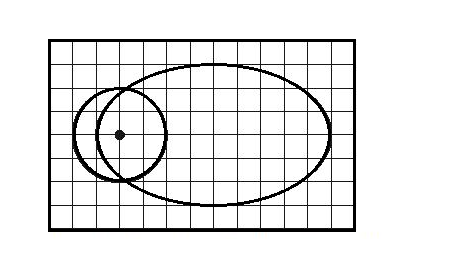# The paths of two small satellites, M1 = 4.00 kg and M2 = 5.00 kg, are shown below, drawn to...

## Question:

The paths of two small satellites, M1 = 4.00 kg and M2 = 5.00 kg, are shown below, drawn to scale, with M1 corresponding to the circular orbit. They orbit around a massive star, also shown below. The orbits are in the plane of the paper. The period of M1 is T1 = 26.0 years. Calculate the period of M2, in years.## Time period of revolution:

When a body revolves around another bigger body in circular/elliptical orbit, the centrifugal force required for motion is provided by the gravitational force between the two bodies.

Become a Study.com member to unlock this answer! Create your account

The time taken to move around in an elliptical orbit depends only on the length of semi major axis a, and is given by {eq}T_{ellipse}^2 = \frac{4\pi^2...Gravitational Pull of the Earth: Definition & Overview

from

Chapter 15 / Lesson 17
30K

Earth's gravitational pull is often misunderstood, but without it, life on Earth would be impossible. In this lesson, we'll define the gravitational pull and give some examples of how it is used. A quiz is provided to test your understanding.